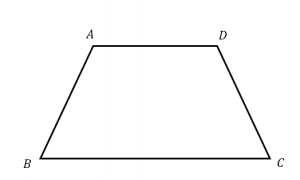# Isosceles Trapezoid Formula

As a quadrilateral, the trapezoid is a four-sided shape. The unique property about the trapezoid is that it has only one pair of parallel sides. Isosceles trapezoid is a type of trapezoid where the non-parallel sides are equal in length.There are two isosceles trapezoid formulas

The Perimeter of isosceles trapezoid formula is

$\large Perimeter\;of\;Isosceles\;Trapeziod=a+b+2c$

Where,
a, b and c are the sides of the trapezoid

The Area of isosceles trapezoid formula is

$\large Area\;of\;Isosceles\;Trapeziod=h\left [\frac{a+b}{2}\right]$

Where,
a and b are the parallel sides of trapezoid.

Solved Examples

Question 1: A trapezoid has legs 8 cm each in length. Its bases are 10 cm and 15 cm respectively. Calculate its perimeter.

Solution:

Given:
a = 10 cm
b = 15 cm
c = 8 cm

Perimeter is given by: p = a + b + 2c
= 10 + 15 + 2

$$\begin{array}{l}\times\end{array}$$
8
= 41 cm.